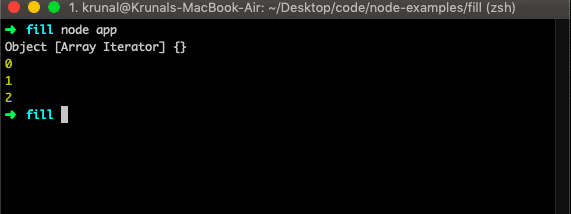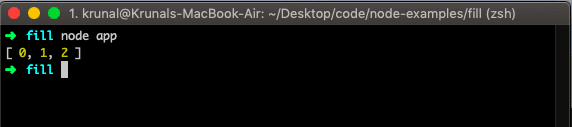# Javascript Array Keys: How to Get Keys of an Array In Javascript

1
116Javascript array keys() method returns the new Array Iterator object that contains the keys for each index in an array. The array keys() method does not change the original array.

## Javascript Array keys()

JavaScript array keys() method returns an Array Iterator object with the keys of an array.

### Syntax

The syntax for the Javascript Array keys() method is the following.

`arr.keys()`

Let us take a simple example.

```// app.js

let arr = ['a', 'b', 'c'];
let iteratorObj = arr.keys();

console.log(iteratorObj);```

So, it has given us iteratorObj. We can get the values by iterating for..of() loop.

```// app.js

let arr = ['a', 'b', 'c'];
let iterator = arr.keys();

console.log(iterator);

for (let key of iterator) {
console.log(key);
}
```We can get the array of keys by the following code.

```// app.js

let arr = ['a', 'b', 'c'];
let iterator = arr.keys();
let keyArray = [];

for (let key of iterator) {
keyArray.push(key);
}
console.log(keyArray);
```If you want to find keys of Object, then check out Javascript Object.keys() example.

## Length of a Javascript associative array

In javascript, there are two types of arrays: The standard arrays and The associative arrays

1. [ ] – standard array – 0 based integer indexes only.
2. { } – associative array – javascript objects where keys can be any strings.

You can define a standard array where indexes can only be integers.

When you do arr[“something”] since something (which is what you use as index) is not an integer, you are defining a property to the arr object (everything is an object in javascript). But you are not adding an element to the standard array.

You can use the array notation to refer to object properties, which is what the solutions in this thread shows. What is created instead is an iterable object?

The items in an associative array are ordered in the order they were declared. With iterable objects, browsers each have their method of sorting the items in an iterable object.

We can not find the length of an associative array or object directly.

So we need to use the Object.keys() function and then use the length property to find the length.

See the following code.

```let shows = new Array();

shows["s1"] = "StrangerThings 1";
shows["s2"] = "StrangerThings 2";
shows["s3"] = "StrangerThings 3";

console.log(Object.keys(shows).length);```

Finally, Javascript Array Keys Tutorial is over.

## Recommended Posts

Javascript Array filter()

Javascript Array map()

Javascript Array reduce()

Javascript Array includes()

Javascript Array forEach()

#### 1 Comment

1.Amit Parmar

thanks for the post

This site uses Akismet to reduce spam. Learn how your comment data is processed.